# Tag: arxiv

A truly good math-story gets spread rather than scrutinized. And a good story it was : more than a millenium before Plato, the Neolithic Scottish Math Society classified the five regular solids : tetrahedron, cube, octahedron, dodecahedron and icosahedron. And, we had solid evidence to support this claim : the NSMS mass-produced stone replicas of their finds and about 400 of them were excavated, most of them in Aberdeenshire.

Six years ago, Michael Atiyah and Paul Sutcliffe arXived their paper Polyhedra in physics, chemistry and geometry, in which they wrote :

Although they are termed Platonic solids there is
convincing evidence that they were known to the Neolithic people of Scotland at least a
thousand years before Plato, as demonstrated by the stone models pictured in ﬁg. 1 which
date from this period and are kept in the Ashmolean Museum in Oxford.

Fig. 1 is the picture below, which has been copied in numerous blog-posts (including my own scottish solids-post) and virtually every talk on regular polyhedra.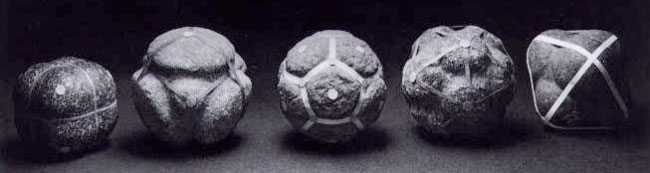From left to right, stone-ball models of the cube, tetrahedron, dodecahedron, icosahedron and octahedron, in which ‘knobs’ correspond to ‘faces’ of the regular polyhedron, as best seen in the central dodecahedral ball.

But then … where’s the icosahedron? The fourth ball sure looks like one but only because someone added ribbons, connecting the centers of the different knobs. If this ribbon-figure is an icosahedron, the ball itself should be another dodecahedron and the ribbons illustrate the fact that icosa- and dodeca-hedron are dual polyhedra. Similarly for the last ball, if the ribbon-figure is an octahedron, the ball itself should be another cube, having exactly 6 knobs.
Who did adorn these artifacts with ribbons, thereby multiplying the number of ‘found’ regular solids by two (the tetrahedron is self-dual)?

The picture appears on page 98 of the book Sacred Geometry (first published in 1979) by Robert Lawlor. He attributes the NSMS-idea to the book Time Stands Still: New Light on Megalithic Science (also published in 1979) by Keith Critchlow. Lawlor writes

The five regular polyhedra or
Platonic solids were known and worked with
well before Plato’s time. Keith Critchlow in
his book Time Stands Still presents convincing
evidence that they were known to the Neolithic peoples of Britain at least 1000 years
before Plato. This is founded on the existence
of a number of sphericalfstones kept in the
Ashmolean Museum at Oxford. Of a size one
can carry in the hand, these stones were carved
into the precise geometric spherical versions of
the cube, tetrahedron, octahedron, icosahedron
and dodecahedron, as well as some additional
compound and semi-regular solids, such as the
cube-octahedron and the icosidodecahedron.
Critchlow says, ‘What we have are objects
clearly indicative of a degree of mathematical
ability so far denied to Neolithic man by any
archaeologist or mathematical historian’. He
speculates on the possible relationship of these
objects to the building of the great astronomical stone circles of the same epoch in Britain:
‘The study of the heavens is, after all, a
spherical activity, needing an understanding of
spherical coordinates. If the Neolithic inhabitants of Scotland had constructed Maes Howe
before the pyramids were built by the ancient
Egyptians, why could they not be studying the
laws of three-dimensional coordinates? Is it not
more than a coincidence that Plato as well as
Ptolemy, Kepler and Al-Kindi attributed
cosmic significance to these figures?’

As Lawlor and Critchlow lean towards mysticism, their claims should not be taken for granted. So, let’s have a look at these famous stones kept in the Ashmolean Museum. The Ashmolean has a page dedicated to their Stone Balls, including the following picture (the Critchlow/Lawlor picture below, for comparison)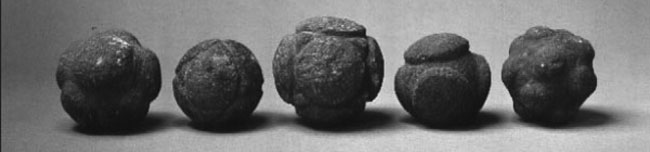The Ashmolean stone balls are from left to right the artifacts with catalogue numbers :

• Stone ball with 7 knobs from Marnoch, Banff (AN1927.2728)
• Stone ball with 6 knobs and isosceles triangles between, from Fyvie, Aberdeenshire (AN1927.2731)
• Stone ball with 6 knobs and isosceles triangles between, from near Aberdeen (AN1927.2730)
• Stone ball with 4 knobs from Auchterless, Aberdeenshire (AN1927.2729)
• Stone ball with 14 knobs from Aberdeen (AN1927.2727)

Ashmolean’s AN 1927.2729 may very well be the tetrahedron and AN 1927.2727 may be used to forge the ‘icosahedron’ (though it has 14 rather than 12 knobs), but the other stones sure look different. In particular, none of the Ashmolean stones has exactly 12 knobs in order to be a dodecahedron.

Perhaps the Ashmolean has a larger collection of Scottish balls and today’s selection is different from the one in 1979? Well, if you have the patience to check all 9 pages of the Scottish Ball Catalogue by Dorothy Marshall (the reference-text when it comes to these balls) you will see that the Ashmolean has exactly those 5 balls and no others!

The sad lesson to be learned is : whether the Critchlow/Lawlor balls are falsifications or fabrications, they most certainly are NOT the Ashmolean stone balls as they claim!

Clearly this does not mean that no neolithic scott could have discovered some regular polyhedra by accident. They made an enormous amount of these stone balls, with knobs ranging from 3 up to no less than 135! All I claim is that this ball-carving thing was more an artistic endeavor, rather than a mathematical one.

There are a number of musea having a much larger collection of these stone balls. The Hunterian Museum has a collection of 29 and some nice online pages on them, including 3D animation. But then again, none of their balls can be a dodecahedron or icosahedron (according to the stone-ball-catalogue).

In fact, more than half of the 400+ preserved artifacts have 6 knobs. The catalogue tells that there are only 8 possible candidates for a Scottish dodecahedron (below their catalogue numbers, indicating for the knowledgeable which museum owns them and where they were found)

• NMA AS 103 : Aberdeenshire
• AS 109 : Aberdeenshire
• AS 116 : Aberdeenshire (prob)
• AUM 159/9 : Lambhill Farm, Fyvie, Aberdeenshire
• Dundee : Dyce, Aberdeenshire
• GAGM 55.96 : Aberdeenshire
• Montrose = Cast NMA AS 26 : Freelands, Glasterlaw, Angus

The case for a Scottish icosahedron looks even worse. Only two balls have exactly 20 knobs

• NMA AS 110 : Aberdeenshire
• GAGM 92 106.1. : Countesswells, Aberdeenshire

Here NMA stands for the National Museum of Antiquities of Scotland in Edinburg (today, it is called ‘National Museums Scotland’) and
GAGM for the Glasgow Art Gallery and Museum. If you happen to be in either of these cities shortly, please have a look and let me know if one of them really is an icosahedron!

UPDATE (April 1st)

Victoria White, Curator of Archaeology at the
Kelvingrove Art Gallery and Museum, confirms that the Countesswells carved stone ball (1892.106.l) has indeed 20 knobs. She gave this additional information :

The artefact came to Glasgow Museums in the late nineteenth century as part of the John Rae collection. John Rae was an avid collector of prehistoric antiquities from the Aberdeenshire area of Scotland. Unfortunately, the ball was not accompanied with any additional information regarding its archaeological context when it was donated to Glasgow Museums. The carved stone ball is currently on display in the ‘Raiders of the Lost Art’ exhibition.

Dr. Alison Sheridan, Head of Early Prehistory, Archaeology Department, National Museums Scotland makes the valid point that new balls have been discovered after the publication of the catalogue, but adds :

Although several balls have turned up since Dorothy Marshall wrote her synthesis, none has 20 knobs, so you can rely on Dorothy’s list.

She has strong reservations against a mathematical interpretation of the balls :

Please also note that the mathematical interpretation of these Late Neolithic objects fails to take into account their archaeological background, and fails to explain why so many do not have the requisite number of knobs! It’s a classic case of people sticking on an interpretation in a state of ignorance. A great shame when so much is known about Late Neolithic archaeology.

Today, Alain Connes and Caterina Consani arXived their new paper Schemes over $\mathbb{F}_1$ and zeta functions. It is a follow-up to their paper On the notion of geometry over $\mathbb{F}_1$, which I’ve tried to explain in a series of posts starting here.

As Javier noted already last week when they updated their first paper, the main point of the first 25 pages of the new paper is to repace abelian groups by abelian monoids in the definition, making it more in tune with other approaches, most notably that of Anton Deitmar. The novelty, if you want, is that they package the two functors $\mathbf{rings} \rightarrow \mathbf{sets}$ and $\mathbf{ab-monoid} \rightarrow \mathbf{sets}$ into one functor $\mathbf{ring-monoid} \rightarrow \mathbf{sets}$ by using the ‘glued category’ $\mathbf{ring-monoid}$ (an idea they attribute to Pierre Cartier).

In general, if you have two categories $\mathbf{cat}$ and $\mathbf{cat’}$ and a pair of adjoint functors between them, then one can form the glued-category $\mathbf{cat-cat’}$ by taking as its collection of objects the disjoint union of the objects of the two categories and by defining the hom-sets between two objects the hom-sets in either category (if both objects belong to the same category) or use the adjoint functors to define the new hom-set when they do not (the very definition of adjoint functors makes that this doesn’t depend on the choice).

Here, one uses the functor $\mathbf{ab-monoid} \rightarrow \mathbf{rings}$ assigning to a monoid $M$ its integral monoid-algebra $\mathbb{Z}[M]$, having as its adjoint the functor $\mathbf{rings} \rightarrow \mathbf{ab-monoid}$ forgetting the additive structure of the commutative ring.

In the second part of the paper, they first prove some nice results on zeta-functions of Noetherian $\mathbb{F}_1$-schemes and extend them, somewhat surprisingly, to settings which do not (yet) fit into the $\mathbb{F}_1$-framework, namely elliptic curves and the hypothetical $\mathbb{F}_1$-curve $\overline{\mathbf{spec}(\mathbb{Z})}$.

Last time I promised to come back explaining how to set-up LaTeX-support, figuring I had to tell you about a few modifications I had to make in order to get Latexrender run on my mac…

A few google searches made it plain how out of touch I am on these matters (details below). But first, there was this comment to this series by Link Starbureiy :

“I took part in Gowers’ blog discussion. My input was to move things over to Google collaboration tools, like Google Knol, and perhaps Google Sites. However, those tools for large-scale collaboration may not be the best solution anymore. I like the NSN idea, but worry about it’s very long-term stability. Would you consider porting the project over to the Google App Engine so that it can be played with in the orkut sandbox (http://sandbox.orkut.com)?”

I thought I made it clear from the outset that I didn’t want to spend the rest of my life web-mastering a site such as NSN. All I wanted to show is that the technology is there free for the taking, and show that you do not have to be a wizard to get it running even on a mac…

I would really love it when some groups, or universities, on institutes, would set up something resembling this dedicated to a single arXiv-topic. Given our history, Antwerp University might be convinced to do this for math.RA but (a) I’m not going to maintain this on my own and (b) there may very well be a bandwidth problem if such a thing would become successful… (although, from past experiences and attempts I’ve made over the years, this is extremely unlikely for this target-group).

So please, if your group has some energy to spare, set-up your own math2.0-network, port it to Google Apps, Knol, Orkut or whatever, and I’d love to join and contribute to it.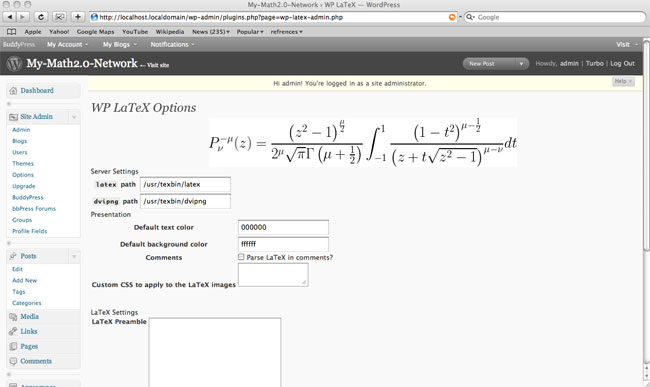Last time I wondered whether a set-up like WordPress.com meets FaceBook with add-ons (such as wiki- and latex-support) might be a usable environment for people working in a specific arXiv-topic.

I’ve used WordPressMU and BuddyPress to create such an embryonal environment. At first I thought I’d extend it a bit before going online but I fail to have the energy right now so I might as well make the link available. If you are into math.QA and/or math.RA you are invited to join the experiment. But, please use this site gently as I’ll have to drop it otherwise.

I’ve no desire to maintain this site indefinitely but would welcome others to set up something similar. For this reason I’ll write a couple of posts explaining how you can build it yourself when you’d have a free afternoon and a spare Mac around. Each post should not take you longer than 1 hour. Today, we’ll provide the boring but essential basics : we must get a MySQL-server and a WebServer running. Next time, I’ll take you through the WordPressMU (MU for multi-users) and BuddyPress installation. After that, we’ll add extra functionality.

We will start from a vanilla 10.5.6 installation. We will often need to edit files, so we’d better grab a good, free  texteditor : TextWrangler, drag it to Applications and place it in the Dock. We’ll also type in commands so we want the TerminalApp (to be found in Applcations/Utilities) in the Dock. SystemPreferences and Safari are already in the Dock and as we will need these tools a lot we might rearrange the Dock to look likeFrom left to right : the Finder, Terminal, Safari, TextWrangler and System Preferences. From now on we will mean by ‘Open …’ that you click on the ‘…’ icon. In the end we want our computer to become a web-server, so we don’t want it to go to sleep. Open SystemPreferences and look for the ‘Energy Saver’-icon, click on the ‘Show details’ button and set the ‘Put the computer to sleep when it is inactive for:’ to Never and unmark the ‘Put the hard disk(s) to sleep when possible’ at the bottom.

We will need to start or stop the WebServer so here’s how that’s done : open SystemPreferences and look for the ‘Sharing’-icon. Marking the ‘Web Sharing’ option is equivalent to starting your webserver (you can verify this by opening Safari and pointing it to http://localhost/ and you should see the default Apache-screen), unmarking it stops the webserver (check this by repeating the previous, now you should get a ‘Safari can’t connect to the server’ message).

All of this was probably trivial to you so let’s do something a bit more advanced : setting up a database-server. OSX doesn’t come with MySql, so we need to download and install it.

MySQL :

Get the latest version : choose the Mac OS X 10.5 (x86)-package and download it (they ask you to register but you can bypass this by clicking on the ‘No thanks, just take me to the downloads’-link). It is a 55.3 Mb file so this may take a couple of minutes. If all goes well this window should pop-up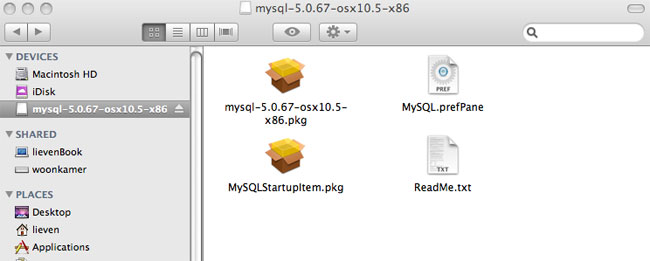Click on the mysql-5.0.67-osx10.5-x86.pkg icon and follow the instruction (defaults suffice, you’ll be asked to give your sudo password and in all it will take less than a minute). Repeat this procedure with MySQLStartupItem.pkg. Done!

To verify it, Open Terminal and type this to the prompt

sudo /Library/StartupItems/MySQLCOM/MySQLCOM start

You’ll get a scary warning message but type in your sudo-password and the Mysql-server will start. You can access it by typing

/usr/local/mysql/bin/mysql

and type exit to the mysql-prompt to leave.
In all, your interaction with the terminal should look something like this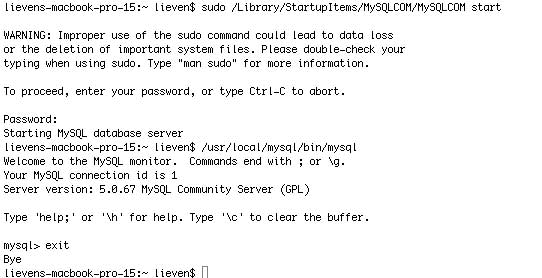Clearly, you do not want to type all of this every time, so we will add the mysql-location to our ‘PATH’. To do this, open TextWrangler and add this line to the blank document

export PATH=$PATH:/usr/local/mysql/bin and save the file as .profile in your home-directory (the one with the ‘House’-icon, usually given your name). You will get a warning that .-files are reserved but go ahead anyway by clicking the use . – button). Now, open Terminal and type this source ~./profile echo$PATH

if all went well you should now see the mysql-location at the end of your path. From now on you’ll only have to type

mysql

to the terminal-prompt to open MySql. At the moment the root-user of your mysql has no password which isnt safe so we’d better set one. Open terminal and type

where, of course, you replace NEWPASSWORD with your choice (use only letters and numbers). From now on you can access your mysql-server by opening Terminal and typing

mysql -u root -p

and giving your password. Okay, so we’ve established our first goal, we have a working Mysql. Take a break if you need one.

better PHP

Mac 10.5 comes equipped with php5 but unfortunately it isn’t quite up to what we need. So, we need to install a better one and tell the mysql-server and the webserver to use the new one instead of the standard one.

Open Safari and grab the better php-version by going to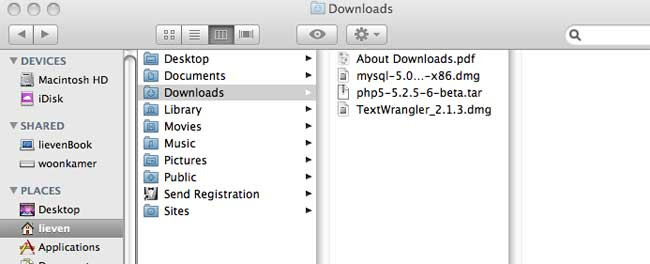Doubleclick on the php5-5.2.5-6-beta.tar file and a new directory will be created called php5. We will now move this directory and lay some symbolic links. Open a new Terminal window and type the following commands (and provide your sudo-password when asked)

sudo mv php5 /usr/local
sudo ln -sf /usr/local/php5/entropy-php.conf /etc/apache2/other/+entropy-php.conf
sudo mkdir /var/mysql
sudo ln -s /tmp/mysql.sock /var/mysql/mysql.sock

Next, we have to tell the webserver to use this new php-version instead of the old one. This information is contained in the apache-configuration file : httpd.conf. Open TextWrangler and under ‘File’ choose the option ‘Open File by Name’. Type /etc/apache2/httpd.conf in the field that appears. The file will now appear in the main window. Under ‘Search’ choose the ‘Go to Line’ option and fill in 114 and hit the Go To button. The follwing line should now be highlighted

immediately under it add the following line (TextWrangler will tell you that the file is owned by root and ask you whether you want to open it, click yes and make the changes)

(observe that line 114 is commented out, that is, starts with a #, whereas your added line is not).

Next, we will have to tell php to communicate with the mysql-server. Again, open TextWrangler, under ‘File’ choose ‘Open File by Name’ and type in /usr/local/php5/lib/php.ini-recommended. When the file appears, under ‘Search’ choose ‘Go to Line’ and type in 810. It will read

mysql.default_socket =

Change it as follows (that is, add to it)

mysql.default_socket = /var/mysql/mysql.sock

and now choose under ‘File’ the ‘Save as…’ option. In the window change php.ini-recommended to php.ini and click Save. Done!

Testing…

Restart your webserver. Recall that this means: open SystemPreferences, choose ‘Sharing’, unmark ‘Web Server’, wait 5 seconds and then mark it again.

Open TextWranger, make a new Text document containing just one line (remove the white space between the ?-signs and the brackets) :

< ?php phpinfo() ? >

Choose ‘File’ and ‘Save as…’ and in the window that appears navigate to YourHardDisk/Library/WebServer/Documents, name the file ‘test.php’ and click the ‘Save’ button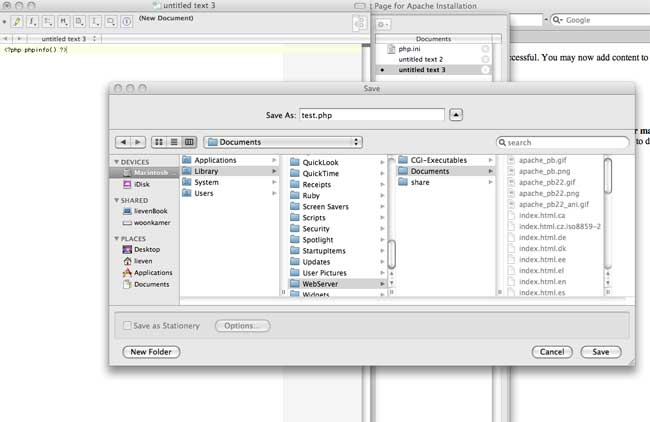Finally, open Safari and point it to http://localhost/test.php. Cross your fingers and if you get a screen like the one below treat yourself to something nice!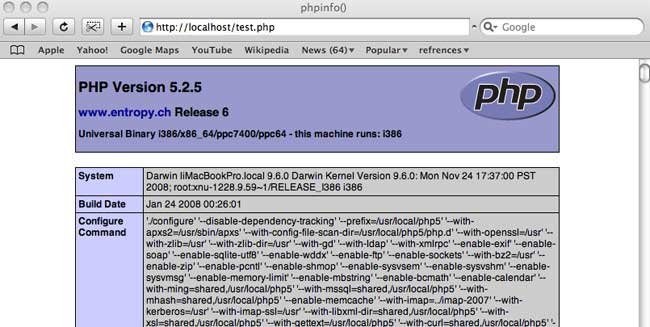At present, some interesting experiments are going on exploring the potential of web 2.0 for mathematical research, that is, setting up a usable math 2.0 – environment.

The starting point is that math 2.0 should be something like blogs+extras. Most mathematicians are not that interested in the latest ICT-tools, but at least they are slowly getting used to reading blogs, so we should stick to this medium and try to enhance it for online-research.

Michael Nielsen has written a couple of posts on this : an after-dinner talk about doing science online aiming at a mathematics audience, building on an essay on the future of science.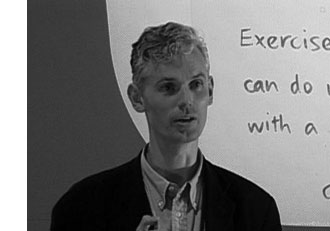Both posts were influential to Tim Gowers‘ dream of massively collaborative mathematics. He took an interesting problem, laid down a set of 12 rules-of-conduct and invited everyone to contribute. The project is still gaining momentum and Terry Tao is also posting about it on his blog.

Michael Nielsen compared Gowers’ approach to long established practice in the open-source software community.

Another interesting experiment is nLab, a knowledge-wiki set up by the reader-community of the n-category cafe. They describe it as : “In other words: this place is like the library, or alchemist’s laboratory, in the back room of the n-Category Café. You come here to work and go there to chat.
We are hoping to create here a space for presentation and archival storage of collaborative work of encyclopedic, didactic, expositional, but also original nature. This will include, but not be limited to, the subjects being discussed every day in the n-Café.”

Both experiments are working great, aided by the authority-status of the blogger, resp. the popularity of the blog, within the research topic. But, what about topics failing to have a blogger or blog of similar status? Should we all drop our current research-interest and convert to either combinatorics or higher-categories?

History taught us in case of failing authority we’d better settle for ‘manageable anarchy’. So, here’s my math2.0-anarchy-allowed-proposal :

• per research-topic (say, an arXiv-topic) we’ll set up a seperate online-reasearch-environment
• anyone interested in that topic is allowed to register and fill-out a profile linking to her list of publications, describe his research interests, her ongoing projects and other trivia
• some may want to start a blog within the environment or join an already existing one, and should be allowed to do so
• some may opt just to read blog posts and occasionally comment, and again, should be allowed to do so
• some may want to set up a research-group to solve a specific problem. they may choose to do this in the open, or as a covert-operation, taking on new members only by invitation
• some may use the environment mainly for networking or chatting-up with their friends
• some research-groups may want to start a group-blog or knowledge-wiki to archive their finds
• surely we’ll be not discussing math in ASCII but in latex
• anyone can see site-wide activity online, see who’s currently there and chat if they feel the need
• anyone can do whatever sensible web2.0-thing there is I forgot by age and hence by ignorance

If this seems like a tall order to satisfy, a bit of research will show that we live at the fortunate moment in time when all the basic ingredient are there, freely available, to do just that!

Over the last weeks I’ve wasted too many hours googling for help, reading-up different fora to get it all working, but … somehow succeeded. Here’s a screen-shot of my very-own NSN (for : noncommutative-social-network) :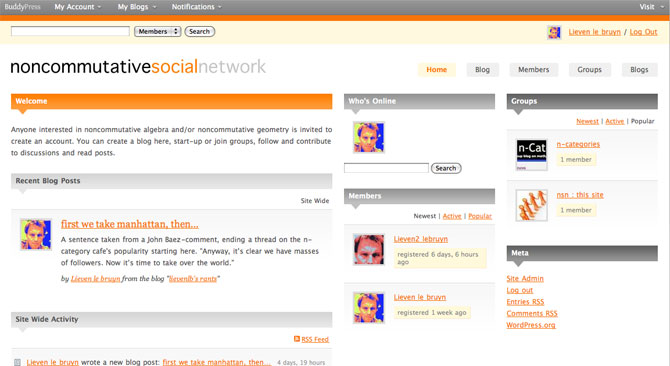Please allow me a few more days to tidy things up and then I’ll make the link available so that anyone interested can experiment with it.

But then, I’ve no desire to spend my days web-mastering such a site. Perhaps some of you would like to take this on, provided you’d get it on a silver plate? (that is, without having to spend too much time setting it up).

So. I’ll run a series of posts explaining how to “set-up your own math2.0 environment”. I’m not aiming at the internet-savvy ones (they’ll probably do it a lot more efficiently), but at people like myself, who are interested to investigate web-based possibilities, but need to be told where to find the very basics, such as the location of their httpd.conf file or their php.ini and such.

I’ve zeroed my MacBookPro, re-installed OSX 10.5 from scratch, upgraded it to current 10.5.6 but no extras (say, vanilla 10.5.6). And I’ll guide you from there, in all gory details, with plenty of screen-shots as I would have liked to find them when I tried to set this up.

Last time we tried to generalize the Connes-Consani approach to commutative algebraic geometry over the field with one element $\mathbb{F}_1$ to the noncommutative world by considering covariant functors

$N~:~\mathbf{groups} \rightarrow \mathbf{sets}$

which over $\mathbb{C}$ resp. $\mathbb{Z}$ become visible by a complex (resp. integral) algebra having suitable universal properties.

However, we didn’t specify what we meant by a complex noncommutative variety (resp. an integral noncommutative scheme). In particular, we claimed that the $\mathbb{F}_1$-‘points’ associated to the functor

$D~:~\mathbf{groups} \rightarrow \mathbf{sets} \qquad G \mapsto G_2 \times G_3$ (here $G_n$ denotes all elements of order $n$ of $G$)

were precisely the modular dessins d’enfants of Grothendieck, but didn’t give details. We’ll try to do this now.

For algebras over a field we follow the definition, due to Kontsevich and Soibelman, of so called “noncommutative thin schemes”. Actually, the thinness-condition is implicit in both Soule’s-approach as that of Connes and Consani : we do not consider R-points in general, but only those of rings R which are finite and flat over our basering (or field).

So, what is a noncommutative thin scheme anyway? Well, its a covariant functor (commuting with finite projective limits)

$\mathbb{X}~:~\mathbf{Alg}^{fd}_k \rightarrow \mathbf{sets}$

from finite-dimensional (possibly noncommutative) $k$-algebras to sets. Now, the usual dual-space operator gives an anti-equivalence of categories

$\mathbf{Alg}^{fd}_k \leftrightarrow \mathbf{Coalg}^{fd}_k \qquad A=C^* \leftrightarrow C=A^*$

so a thin scheme can also be viewed as a contra-variant functor (commuting with finite direct limits)

$\mathbb{X}~:~\mathbf{Coalg}^{fd}_k \rightarrow \mathbf{Sets}$

In particular, we are interested to associated to any {tex]k $-algebra$A $its representation functor :$\mathbf{rep}(A)~:~\mathbf{Coalg}^{fd}_k \rightarrow \mathbf{Sets} \qquad C \mapsto Alg_k(A,C^*) $This may look strange at first sight, but$C^* $is a finite dimensional algebra and any$n $-dimensional representation of$A $is an algebra map$A \rightarrow M_n(k) $and we take$C $to be the dual coalgebra of this image. Kontsevich and Soibelman proved that every noncommutative thin scheme$\mathbb{X} $is representable by a$k $-coalgebra. That is, there exists a unique coalgebra$C_{\mathbb{X}} $(which they call the coalgebra of ‘distributions’ of$\mathbb{X} $) such that for every finite dimensional$k $-algebra$B $we have$\mathbb{X}(B) = Coalg_k(B^*,C_{\mathbb{X}}) $In the case of interest to us, that is for the functor$\mathbf{rep}(A) $the coalgebra of distributions is Kostant’s dual coalgebra$A^o $. This is the not the full linear dual of$A $but contains only those linear functionals on$A $which factor through a finite dimensional quotient. So? You’ve exchanged an algebra$A $for some coalgebra$A^o $, but where’s the geometry in all this? Well, let’s look at the commutative case. Suppose$A= \mathbb{C}[X] $is the coordinate ring of a smooth affine variety$X $, then its dual coalgebra looks like$\mathbb{C}[X]^o = \oplus_{x \in X} U(T_x(X)) $the direct sum of all universal (co)algebras of tangent spaces at points$x \in X $. But how do we get the variety out of this? Well, any coalgebra has a coradical (being the sun of all simple subcoalgebras) and in the case just mentioned we have$corad(\mathbb{C}[X]^o) = \oplus_{x \in X} \mathbb{C} e_x $so every point corresponds to a unique simple component of the coradical. In the general case, the coradical of the dual coalgebra$A^o $is the direct sum of all simple finite dimensional representations of$A $. That is, the direct summands of the coalgebra give us a noncommutative variety whose points are the simple representations, and the remainder of the coalgebra of distributions accounts for infinitesimal information on these points (as do the tangent spaces in the commutative case). In fact, it was a surprise to me that one can describe the dual coalgebra quite explicitly, and that$A_{\infty} $-structures make their appearance quite naturally. See this paper if you’re in for the details on this. That settles the problem of what we mean by the noncommutative variety associated to a complex algebra. But what about the integral case? In the above, we used extensively the theory of Kostant-duality which works only for algebras over fields… Well, not quite. In the case of$\mathbb{Z} $(or more general, of Dedekind domains) one can repeat Kostant’s proof word for word provided one takes as the definition of the dual$\mathbb{Z} $-coalgebra of an algebra (which is$\mathbb{Z} $-torsion free)$A^o = { f~:~A \rightarrow \mathbb{Z}~:~A/Ker(f)~\text{is finitely generated and torsion free}~} $(over general rings there may be also variants of this duality, as in Street’s book an Quantum groups). Probably lots of people have come up with this, but the only explicit reference I have is to the first paper I’ve ever written. So, also for algebras over$\mathbb{Z} $we can define a suitable noncommutative integral scheme (the coradical approach accounts only for the maximal ideals rather than all primes, but somehow this is implicit in all approaches as we consider only thin schemes). Fine! So, we can make sense of the noncommutative geometrical objects corresponding to the group-algebras$\mathbb{C} \Gamma $and$\mathbb{Z} \Gamma $where$\Gamma = PSL_2(\mathbb{Z}) $is the modular group (the algebras corresponding to the$G \mapsto G_2 \times G_3 $-functor). But, what might be the points of the noncommutative scheme corresponding to$\mathbb{F}_1 \Gamma $??? Well, let’s continue the path cut out before. “Points” should correspond to finite dimensional “simple representations”. Hence, what are the finite dimensional simple$\mathbb{F}_1 $-representations of$\Gamma $? (Or, for that matter, of any group$G $) Here we come back to Javier’s post on this : a finite dimensional$\mathbb{F}_1 $-vectorspace is a finite set. A$\Gamma $-representation on this set (of n-elements) is a group-morphism$\Gamma \rightarrow GL_n(\mathbb{F}_1) = S_n $hence it gives a permutation representation of$\Gamma $on this set. But then, if finite dimensional$\mathbb{F}_1 $-representations of$\Gamma $are the finite permutation representations, then the simple ones are the transitive permutation representations. That is, the points of the noncommutative scheme corresponding to$\mathbb{F}_1 \Gamma $are the conjugacy classes of subgroups$H \subset \Gamma $such that$\Gamma/H $is finite. But these are exactly the modular dessins d’enfants introduced by Grothendieck as I explained a while back elsewhere (see for example this post and others in the same series). A couple of weeks ago, Alain Connes and Katia Consani arXived their paper “On the notion of geometry over$\mathbb{F}_1 $”. Their subtle definition is phrased entirely in Grothendieck‘s scheme-theoretic language of representable functors and may be somewhat hard to get through if you only had a few years of mathematics.I’ll try to give the essence of their definition of an affine scheme over$\mathbb{F}_1 $(and illustrate it with an example) in a couple of posts. All you need to know is what a finite Abelian group is (if you know what a cyclic group is that’ll be enough) and what a commutative algebra is. If you already know what a functor and a natural transformation is, that would be great, but we’ll deal with all that abstract nonsense when we’ll need it. So take two finite Abelian groups A and B, then a group-morphism is just a map$f~:~A \rightarrow B $preserving the group-data. That is, f sends the unit element of A to that of B and f sends a product of two elements in A to the product of their images in B. For example, if$A=C_n $is a cyclic group of order n with generator g and$B=C_m $is a cyclic group of order m with generator h, then every groupmorphism from A to B is entirely determined by the image of g let’s say that this image is$h^i $. But, as$g^n=1 $and the conditions on a group-morphism we must have that$h^{in} = (h^i)^n = 1 $and therefore m must divide i.n. This gives you all possible group-morphisms from A to B. They are plenty of finite abelian groups and many group-morphisms between any pair of them and all this stuff we put into one giant sack and label it$\mathbf{abelian} $. There is another, even bigger sack, which is even simpler to describe. It is labeled$\mathbf{sets} $and contains all sets as well as all maps between two sets. Right! Now what might be a map$F~:~\mathbf{abelian} \rightarrow \mathbf{sets} $between these two sacks? Well, F should map any abelian group A to a set F(A) and any group-morphism$f~:~A \rightarrow B $to a map between the corresponding sets$F(f)~:~F(A) \rightarrow F(B) $and do all of this nicely. That is, F should send compositions of group-morphisms to compositions of the corresponding maps, and so on. If you take a pen and a piece of paper, you’re bound to come up with the exact definition of a functor (that’s what F is called). You want an example? Well, lets take F to be the map sending an Abelian group A to its set of elements (also called A) and which sends a groupmorphism$A \rightarrow B $to the same map from A to B. All F does is ‘forget’ the extra group-conditions on the sets and maps. For this reason F is called the forgetful functor. We will denote this particular functor by$\underline{\mathbb{G}}_m $, merely to show off. Luckily, there are lots of other and more interesting examples of such functors. Our first class we will call maxi-functors and they are defined using a finitely generated$\mathbb{C} $-algebra R. That is, R can be written as the quotient of a polynomial algebra$R = \frac{\mathbb{C}[x_1,\ldots,x_d]}{(f_1,\ldots,f_e)} $by setting all the polynomials$f_i $to be zero. For example, take R to be the ring of Laurant polynomials$R = \mathbb{C}[x,x^{-1}] = \frac{\mathbb{C}[x,y]}{(xy-1)} $Other, and easier, examples of$\mathbb{C} $-algebras is the group-algebra$\mathbb{C} A $of a finite Abelian group A. This group-algebra is a finite dimensional vectorspace with basis$e_a $, one for each element$a \in A $with multiplication rule induced by the relations$e_a.e_b = e_{a.b} $where on the left-hand side the multiplication . is in the group-algebra whereas on the right hand side the multiplication in the index is that of the group A. By choosing a different basis one can show that the group-algebra is really just the direct sum of copies of$\mathbb{C} $with component-wise addition and multiplication$\mathbb{C} A = \mathbb{C} \oplus \ldots \oplus \mathbb{C} $with as many copies as there are elements in the group A. For example, for the cyclic group$C_n $we have$\mathbb{C} C_n = \frac{\mathbb{C}[x]}{(x^n-1)} = \frac{\mathbb{C}[x]}{(x-1)} \oplus \frac{\mathbb{C}[x]}{(x-\zeta)} \oplus \frac{\mathbb{C}[x]}{(x-\zeta^2)} \oplus \ldots \oplus \frac{\mathbb{C}[x]}{(x-\zeta^{n-1})} = \mathbb{C} \oplus \mathbb{C} \oplus \mathbb{C} \oplus \ldots \oplus \mathbb{C} $The maxi-functor asociated to a$\mathbb{C} $-algebra R is the functor$\mathbf{maxi}(R)~:~\mathbf{abelian} \rightarrow \mathbf{sets} $which assigns to a finite Abelian group A the set of all algebra-morphism$R \rightarrow \mathbb{C} A $from R to the group-algebra of A. But wait, you say (i hope), we also needed a functor to do something on groupmorphisms$f~:~A \rightarrow B $. Exactly, so to f we have an algebra-morphism$f’~:~\mathbb{C} A \rightarrow \mathbb{C}B $so the functor on morphisms is defined via composition$\mathbf{maxi}(R)(f)~:~\mathbf{maxi}(R)(A) \rightarrow \mathbf{maxi}(R)(B) \qquad \phi~:~R \rightarrow \mathbb{C} A \mapsto f’ \circ \phi~:~R \rightarrow \mathbb{C} A \rightarrow \mathbb{C} B $So, what is the maxi-functor$\mathbf{maxi}(\mathbb{C}[x,x^{-1}] $? Well, any$\mathbb{C} $-algebra morphism$\mathbb{C}[x,x^{-1}] \rightarrow \mathbb{C} A $is fully determined by the image of$x $which must be a unit in$\mathbb{C} A = \mathbb{C} \oplus \ldots \oplus \mathbb{C} $. That is, all components of the image of$x $must be non-zero complex numbers, that is$\mathbf{maxi}(\mathbb{C}[x,x^{-1}])(A) = \mathbb{C}^* \oplus \ldots \oplus \mathbb{C}^* $where there are as many components as there are elements in A. Thus, the sets$\mathbf{maxi}(R)(A) $are typically huge which is the reason for the maxi-terminology. Next, let us turn to mini-functors. They are defined similarly but this time using finitely generated$\mathbb{Z} $-algebras such as$S=\mathbb{Z}[x,x^{-1}] $and the integral group-rings$\mathbb{Z} A $for finite Abelian groups A. The structure of these inegral group-rings is a lot more delicate than in the complex case. Let’s consider them for the smallest cyclic groups (the ‘isos’ below are only approximations!)$\mathbb{Z} C_2 = \frac{\mathbb{Z}[x]}{(x^2-1)} = \frac{\mathbb{Z}[x]}{(x-1)} \oplus \frac{\mathbb{Z}[x]}{(x+1)} = \mathbb{Z} \oplus \mathbb{Z} \mathbb{Z} C_3 = \frac{\mathbb{Z}[x]}{(x^3-1)} = \frac{\mathbb{Z}[x]}{(x-1)} \oplus \frac{\mathbb{Z}[x]}{(x^2+x+1)} = \mathbb{Z} \oplus \mathbb{Z}[\rho] \mathbb{Z} C_4 = \frac{\mathbb{Z}[x]}{(x^4-1)} = \frac{\mathbb{Z}[x]}{(x-1)} \oplus \frac{\mathbb{Z}[x]}{(x+1)} \oplus \frac{\mathbb{Z}[x]}{(x^2+1)} = \mathbb{Z} \oplus \mathbb{Z} \oplus \mathbb{Z}[i] $For a$\mathbb{Z} $-algebra S we can define its mini-functor to be the functor$\mathbf{mini}(S)~:~\mathbf{abelian} \rightarrow \mathbf{sets} $which assigns to an Abelian group A the set of all$\mathbb{Z} $-algebra morphisms$S \rightarrow \mathbb{Z} A $. For example, for the algebra$\mathbb{Z}[x,x^{-1}] $we have that$\mathbf{mini}(\mathbb{Z} [x,x^{-1}]~(A) = (\mathbb{Z} A)^* $the set of all invertible elements in the integral group-algebra. To study these sets one has to study the units of cyclotomic integers. From the above decompositions it is easy to verify that for the first few cyclic groups, the corresponding sets are$\pm C_2, \pm C_3 $and$\pm C_4 $. However, in general this set doesn’t have to be finite. It is a well-known result that the group of units of an integral group-ring of a finite Abelian group is of the form$(\mathbb{Z} A)^* = \pm A \times \mathbb{Z}^{\oplus r} $where$r = \frac{1}{2}(o(A) + 1 + n_2 -2c) $where$o(A) $is the number of elements of A,$n_2 $is the number of elements of order 2 and c is the number of cyclic subgroups of A. So, these sets can still be infinite but at least they are a lot more manageable, explaining the mini-terminology. Now, we would love to go one step deeper and define nano-functors by the same procedure, this time using finitely generated algebras over$\mathbb{F}_1 $, the field with one element. But as we do not really know what we might mean by this, we simply define a nano-functor to be a subfunctor of a mini-functor, that is, a nano-functor N has an associated mini-functor$\mathbf{mini}(S) $such that for all finite Abelian groups A we have that$N(A) \subset \mathbf{mini}(S)(A) $. For example, the forgetful functor at the beginning, which we pompously denoted$\underline{\mathbb{G}}_m $is a nano-functor as it is a subfunctor of the mini-functor$\mathbf{mini}(\mathbb{Z}[x,x^{-1}]) $. Now we are allmost done : an affine$\mathbb{F}_1 $-scheme in the sense of Connes and Consani is a pair consisting of a nano-functor N and a maxi-functor$\mathbf{maxi}(R) $such that two rather strong conditions are satisfied : • there is an evaluation ‘map’ of functors$e~:~N \rightarrow \mathbf{maxi}(R) $• this pair determines uniquely a ‘minimal’ mini-functor$\mathbf{mini}(S) $of which N is a subfunctor of course we still have to turn this into proper definitions but that will have to await another post. For now, suffice it to say that the pair$~(\underline{\mathbb{G}}_m,\mathbf{maxi}(\mathbb{C}[x,x^{-1}])) $is a$\mathbb{F}_1 $-scheme with corresponding uniquely determined mini-functor$\mathbf{mini}(\mathbb{Z}[x,x^{-1}]) $, called the multiplicative group scheme. Continued here It is always great to hear about new and clever ways to use blogs and the internet to promote (and hopefully do) science better. So, I’m a keen consumer of the Flash-presentations of the talks at the Science in the 21st century conference. Bee of Backreaction is one of the organizers and has a post on it as does Woit of Not Even Wrong. Chad Orzel of Uncertain Principles gave an entertaining talk titled Talking to My Dog about Science: Weblogs and Public Outreach. Not that much about the dog bit except that two of his blog-posts explaining physics to his dog landed him a book contract (book scheduled to appear early 2009).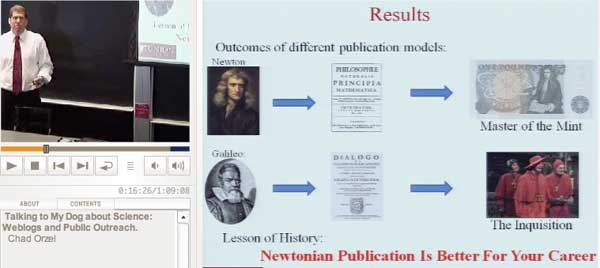He compared two ways of communicating scientific discoveries : the Newtonian way (aka publishing in peer reviewed journals) aiming deliberately to make your texts only readable to the experts, versus the Galileian way (aka blogging or science-journalism) trying to find a method to maximize your readership and concluded (based on history) that the Newton-manner is far better for your career… Jacques Distler of Musings continued his crusade to convince us to use mathML for TeX-rendering in Blogs, Wikis, MathML: Scientific Communication. Of course he is right, but as long as the rendering depends on the client to install extra fonts I’m not going to spend another two weeks sanitizing this blog to make it XHTML-compliant. We’ll just have to wait for html5 and compatible browsers… A talk I found extremely interesting was The Future is a Foreign Country by Timo Hannay of the Nature Publishing Group on the new challenges facing publishers in times of internet.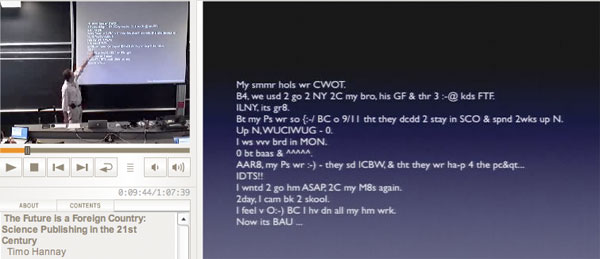Above a text-message filed in as homework (‘describe your holiday’). When Timo decrypted it, I had to think about my old idea of writing a course using only text-messages… Truly shocked was I when I saw the diagram below in Paul Ginsparg’s talk Next-Generation Implications of Open Access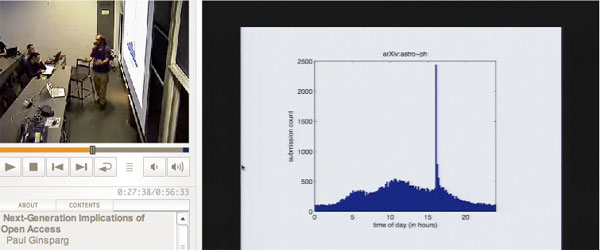It depicts the number of submissions to the arXiv by day-time of submission over 24hours. I would have expected a somewhat smooth pattern but was totally blown away by the huge peak around 16hrs. I’ll let you discover the mystery for yourself but it seems to be related to the dead-line for submission, the corresponding order the papers are mentioned in the emails send out, and its effect on the number of references these papers get within the first year… Somewhat unlucky was Victor Henning in his talk Mendeley: A Last.fm for Research? when he wanted to demonstrate the mendeley web-interface but lost his internet connection…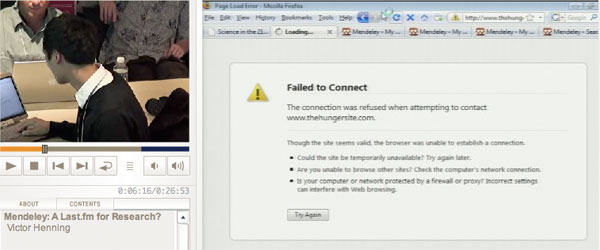Still, it seems like a good initiative so I’ve registered with the mendeley site, downloaded the software and hope to explore it over the coming days. I really hope this will turn out to be the one web2-idea catching on among the mathematics-community…Amidst all LHC-noise, Yuri I. Manin arXived today an interesting paper Cyclotomy and analytic geometry over$\mathbb{F}_1 $. The paper gives a nice survey of the existent literature and focusses on the crucial role of roots of unity in the algebraic geometry over the non-existent field with one element$\mathbb{F}_1 $(in French called ‘F-un’). I have tried to do a couple of posts on F-un some time ago but now realize, reading Manin’s paper, I may have given up way too soon… At several places in the paper, Manin hints at a possible noncommutative geometry over$\mathbb{F}_1 $: This is the appropriate place to stress that in a wider context of Toen-Vaqui ‘Au-dessous de Spec Z’, or eventually in noncommutative$\mathbb{F}_1 $-geometry, teh spectrum of$\mathbb{F}_1 $loses its privileged position as a final object of a geometric category. For example, in noncommutative geometry, or in an appropriate category of stacks, the quotient of this spectrum modulo the trivial action of a group must lie below this spectrum. Soule’s algebras$\mathcal{A}_X $are a very important element of the structure, in particular, because they form a bridge to Arakelov geometry. Soule uses concrete choices of them in order to produce ‘just right’ supply of morphisms, without a priori constraining these choices formally. In this work, we use these algebras and their version also to pave a way to the analytic (and possibly non-commutative) geometry over$\mathbb{F}_1 $. Back when I was writing the first batch of F-un posts, I briefly contemplated the possibility of a noncommutative geometry over$\mathbb{F}_1 $, but quickly forgot about it because I thought it would be forced to reduce to commutative geometry. Here is the quick argument : noncommutative geometry is really the study of coalgebras (see for example my paper or if you prefer more trustworthy sources the Kontsevich-Soibelman paper). Now, unless I made a mistake, I think all coalgebras over$\mathbb{F}_1 \$ must be co-commutative (even group-like), so reducing to commutative geometry.

Surely, I’m missing something…

Over the last month a pile of books grew in our living room to impressive heights, intended to be packed for our usual 3+week vacation to the south of France. From the outset it was clear that ‘circumstances’ (see title for hint) forced us to slim it down to 2 weeks-max, this year.

So, last week I did divide the pile into two, those books I really wanted to read on vacation and those that could wait a bit longer. But then, a few days ago, the bigC stroke again, making it imperative to change our plans (and probably forget about vacation at all, this year). There’s a slim chance we’ll get away for a couple of days, so I made a further selection, just in case.

Below, I’ll give the original list (as well as their fate in the selection process) hoping that you can take them all with you, that is, if life treats your loved ones gentler…

In the category physics-general public books :

In the category mathematics-general public books :

In the category mathematics :

In the category literature :

In the category litter-ature :